#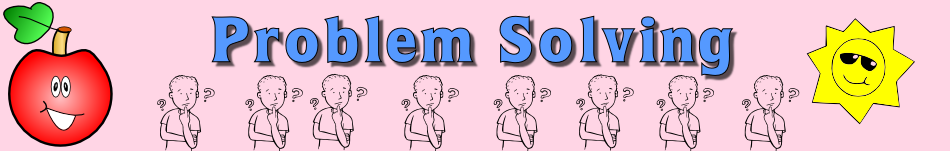There are 366 different Starters of The Day, many to choose from. You will find below some starters on the topic of Problem Solving. A lesson starter does not have to be on the same topic as the main part of the lesson or the topic of the previous lesson. It is often very useful to revise or explore other concepts by using a starter based on a totally different area of Mathematics.

Main Page

### Problem Solving Starters:Add up a sequence of consecutive numbers. Can you find a quick way to do it?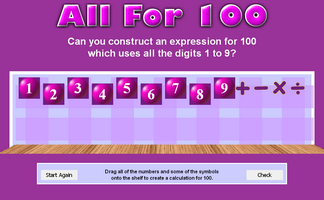Can you write an expression for 100 which uses all the digits 1 to 9?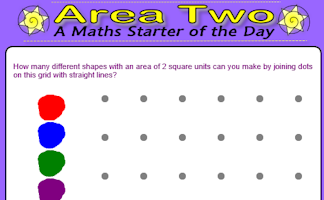How many different shapes with an area of 2 square units can you make by joining dots on this grid with straight lines?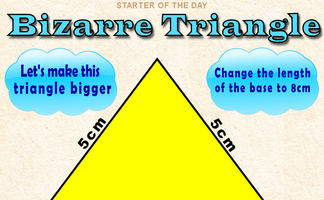By how much would the area of this triangle increase if its base was enlarged to 8cm?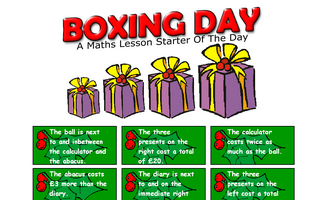Work out the contents and the cost of the Christmas boxes from the given cluesUse only the 1, 5 and 0 keys on a calculator to make given totals.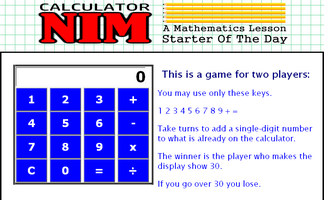A game in which players take turns to add a single-digit number to what is already in the calculator. The winner is the player who makes the display show 30.Calculate the total cost of four cars from the information given.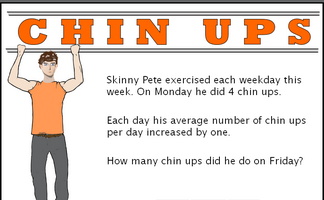Work out the number of chin ups the characters do on the last day of the week give information about averages.Work out the total cost of five Christmas presents from the information given.Can you use the digits on the left of this clock along with any mathematical operations to equal the digits on the right?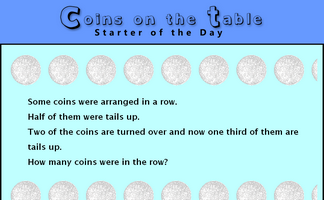A puzzle about the number of coins on a table given information about fractions of them.Three consecutive numbers multiplied together give a given product. Pupils are asked to figure out what the numbers are.Which three consecutive numbers multiplied together give the given answer.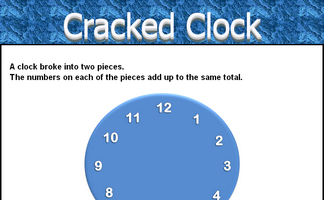How did the clock break if the numbers on each of the pieces added up to the same total?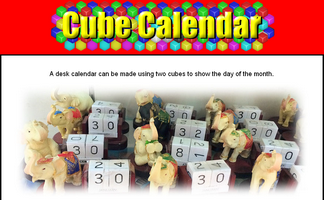What numbers should be on each face of the two cubes to make this perpetual calendar?Determine whether the given nets would fold to produce a dice.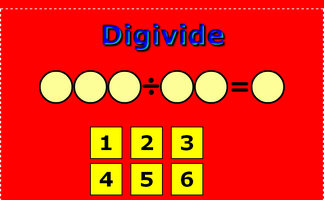Arrange the digits 1 to 6 to make a three digit number divided by a two digit number giving a one digit answer.Fit families onto eleven seater buses without splitting up the families.Find out which of the calculator keys is faulty from the given information. A mathematical puzzle requiring good problem solving strategies.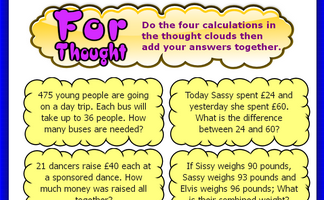Add up the answers to the four real life questions.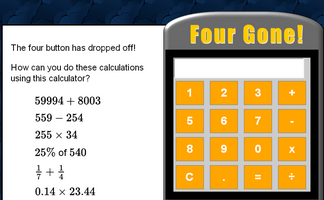An activity involving a broken calculator which is missing the four button. Can you evaluate the given expressions without using the four?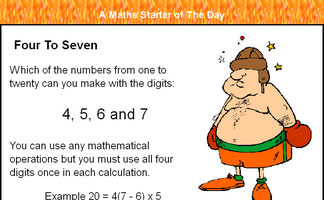Which of the numbers from one to twenty can you make with the digits 4, 5, 6 and 7?A clock face containing only the number 4. Can you make a clock face containing any other single number?Find symmetric words in this ancient cipher.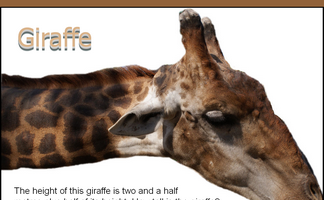The height of this giraffe is three and a half metres plus half of its height. How tall is the giraffe?If all the students in this room shook hands with each other, how many handshakes would there be altogether?If six girls can plant 90 trees in a day. How many trees can ten girls plant in a day? The unitary method.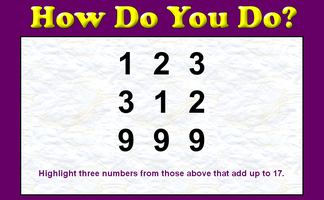A little lateral thinking will help you solve this number puzzle.How many Triangles can you find in the diagram?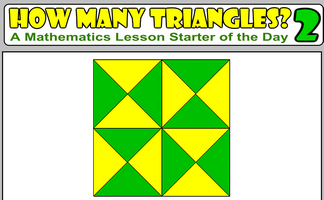How many triangles are hidden in the pattern? What strategy might you use to count them all to ensure you don't miss any out?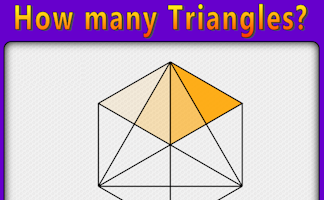Find a systematic way of counting the number of triangles in the given diagram.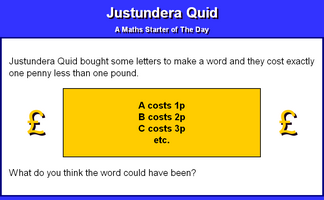Find a word whose letters would cost exactly ninety nine pence.Find the weight of one cuboid (by division) of each colour then add your answers together.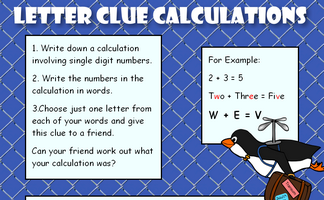Work out what the calculations might be from the letter clues.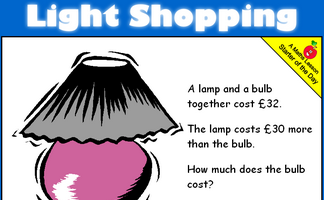A lamp and a bulb together cost 32 pounds. The lamp costs 30 pounds more than the bulb. How much does the bulb cost?A classic matchstick puzzle designed to challenge your spacial awareness.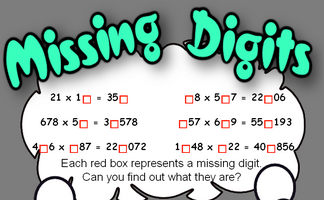Work out what the missing digits in the calculations are.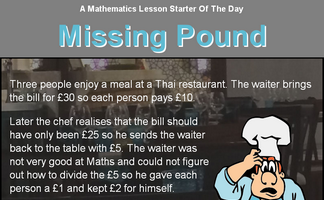A puzzle about a restaurant bill. Exactly where did the missing pound go?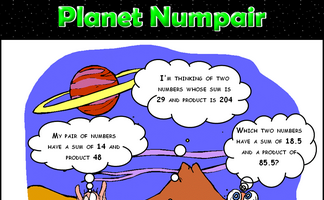Arrange the digits one to nine to make a correct addition calculation.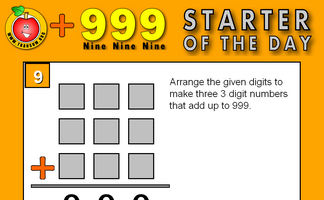Arrange the numbers 1-9 to make three 3 digit numbers that add up to 999.This activity requires eight students to sit non consecutively on a grid of chairs.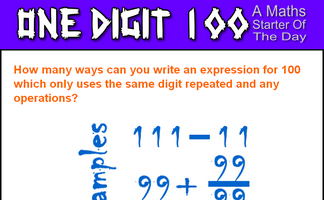How many ways can you write an expression for 100 which only uses the same digit repeated and any operations?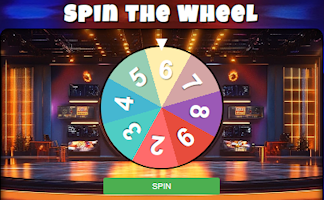Work out the least amount of time for four people to walk through a tunnel?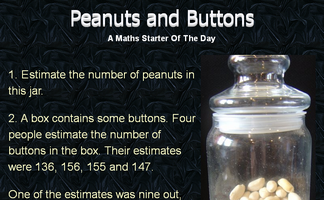Two questions involving estimating a quantity.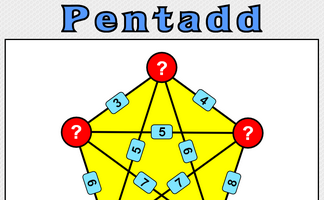Five numbers are added together in pairs and the sums shown. What might the five numbers be?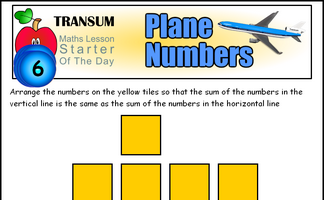Arrange numbers on the plane shaped grid to produce the given totalsArrange the numbers to produce the largest product.Arrange numbers at the bottom of the pyramid which will give the largest total at the top.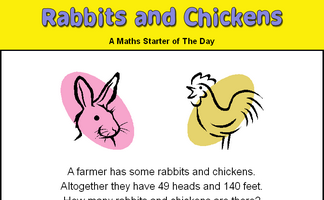There are some rabbits and chickens in a field. Calculate how many of each given the number of heads and feet.Record the weights of the trains to work out the weight of a locomotive and a coach. A real situation which produces simultaneous equations.Find a calculation for the current year which uses all of the digits 1 to 9.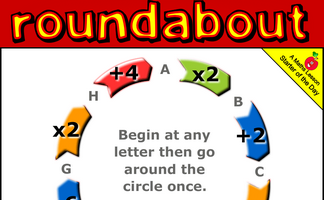Go around the roundabout performing each of the operations. Which starting point gives the largest answer?Can you draw 4 straight lines, without taking your pencil off the paper, which pass through all 9 roses?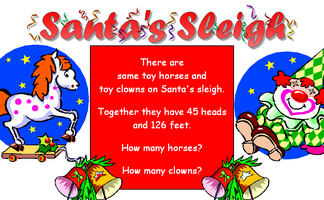Work out the number of clowns and horses given the number of heads and feet.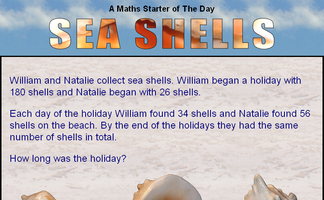A question which can be best answered by using algebra.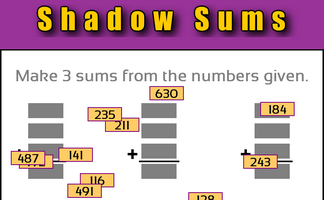Make sums from the three digit numbers given.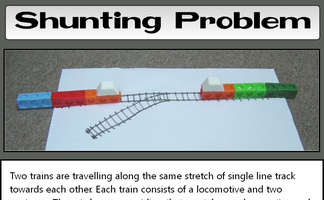Allow two trains to pass by using the limited amount of siding space.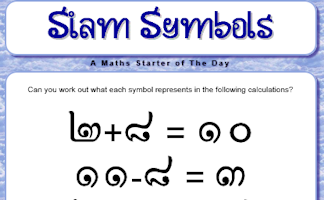Can you work out what each of the strange symbols represents in these calculations?The classic game of Nim played with a group of pens and pencils. The game can be extended to the multi-pile version.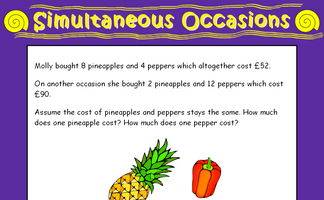A problem which can best be solved as a pair of simultaneous equations.An activity involving a calculator which is missing the six button. Can you evaluate the given expressions without using the six?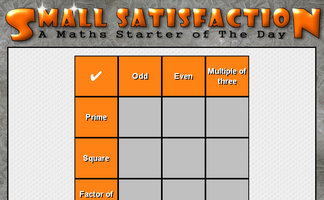Arrange the digits one to nine in the grid so that they obey the row and column headings.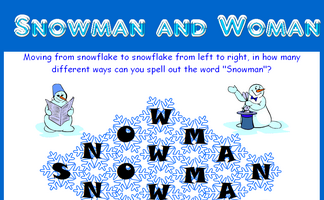How many different ways can you spell out the word "Snowman" by moving from snowflake to snowflake.Arrange the numbers on the grid of squares so that the totals along each line of three squares are equal.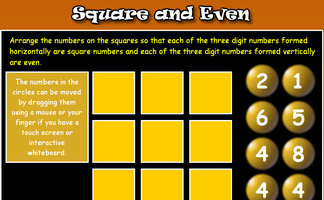Arrange the numbers on the cards so that each of the three digit numbers formed horizontally are square numbers and each of the three digit numbers formed vertically are even.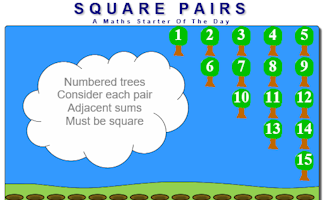Arrange the numbered trees so that adjacent sums are square numbers.Separate three rows of three animals using three squares.Use the information implied in the diagram to calculate the perimeter of this shape.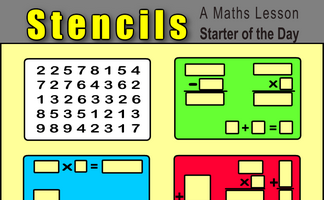Which of the coloured stencils will fit over the numbered card to produce correct calculations?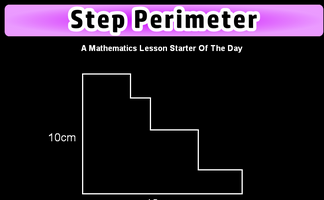Is it possible to work out the perimeter of this shape if not all the side lengths are given?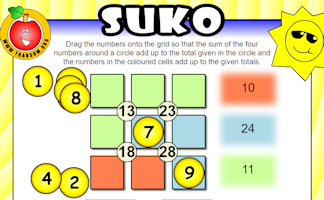Interactive number-based logic puzzle similar to those featuring in The Times and Telegraph newspapers.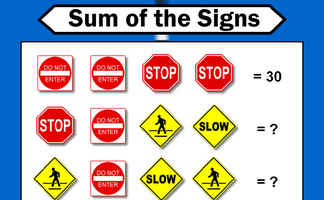Each traffic sign stands for a number. Some of the sums of rows and columns are shown. What numbers might the signs stand for?Arrange the numbers one to eight into the calculations to make the totals correct..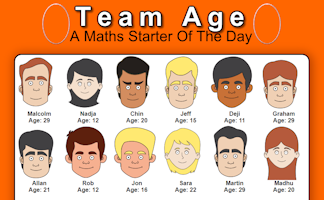Work out who is in which team from the information given.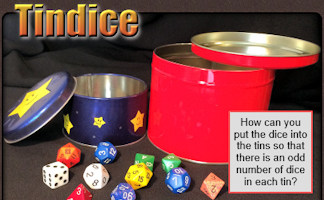How can you put the dice into the tins so that there is an odd number of dice in each tin?In how many different ways might Tran decide to wear his hats in one week?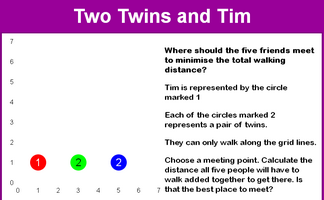A tricky problem set on a coordinate grid.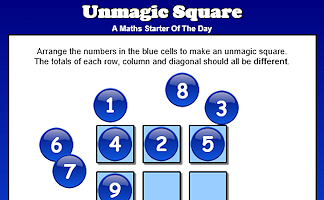Arrange the numbers 1 to 9 in a 3 by 3 grid so that none of the line totals are the same.Each letter stands for a different digit. Can you make sense of word sum?

Advanced Problem Solving Starters

### Search

The activity you are looking for may have been classified in a different way from the way you were expecting. You can search the whole of Transum Maths by using the box below.

Have today's Starter of the Day as your default homepage. Copy the URL below then select
Tools > Internet Options (Internet Explorer) then paste the URL into the homepage field.

Set as your homepage (if you are using Internet Explorer)

Do you have any comments? It is always useful to receive feedback and helps make this free resource even more useful for those learning Mathematics anywhere in the world. Click here to enter your comments.For All: